Author: Oscar Cronquist Article last updated on January 26, 2023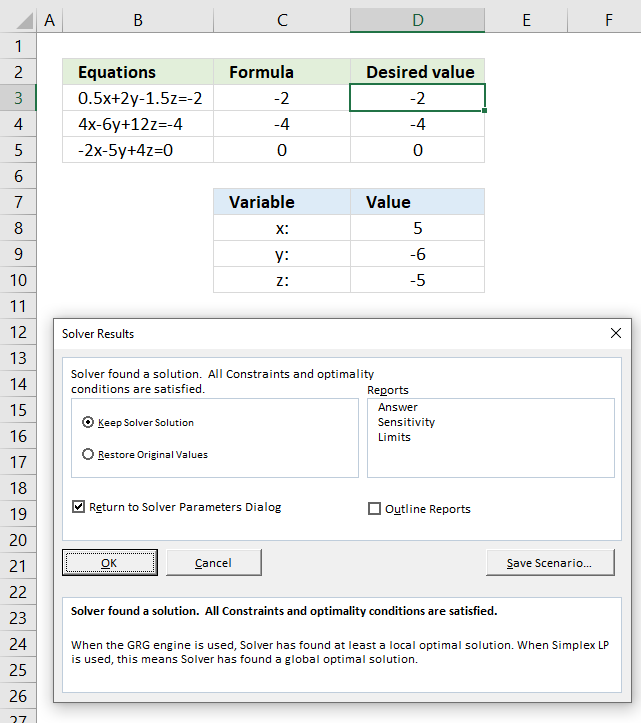This article demonstrates how to solve simultaneous linear equations using formulas and Solver. The variables have the same value in all equations, they are called simultaneous because all variables are equal in all equations.

## How to convert equations to standard form?

The examples below are easier to follow if you convert the equations to standard form. This means that the variables are on the left side and the constant is on the right side of the equation.

ax + by + cz = d

Example, the following equation is not standard form:

4 - 1.5z = -0.5x - 2y + 2

To move the variables on the right side to the left side add or subtract the variables based on their sign on both sides.

4 - 1.5z = -0.5x - 2y + 2

becomes

+0.5x + 2y + 4 - 1.5z = -0.5x - 2y + 0.5x + 2y + 2

becomes

0.5x + 2y - 1.5z + 4 = 2

Now do the same thing with the constants, however, move them to the right side of the equation.

0.5x + 2y - 1.5z + 4 = 2

becomes

0.5x + 2y - 1.5z + 4 - 4 = 2 - 4

becomes

0.5x + 2y - 1.5z = -2

## 1. Solve linear equations using Solver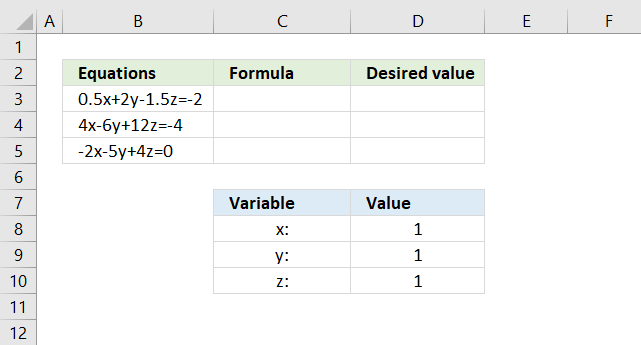The image above shows three equations in cell range B3:B5, each equation contains three variables x, y and z.

We can create these equations as formulas if we use named ranges as variables. We will create three named ranges for cells D8, D9 and D10. Here are the steps described in detail.

1. Select cell D8.
2. Press with left mouse button on in the name bar.
3. Type x in the name bar, see image below.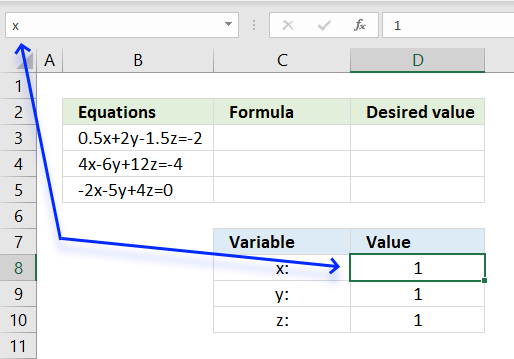4. Press Enter to name cell D8 x.

Repeat above steps with cell D9 and D10 using names y and z respectively. We can now use these named ranges in our formulas. We need to rewrite the equations so Excel can interpret them. The asterisk character multiplies a number with a named range.

Formula in cell C3:

=0.5*x+2*y-1.5*z

Formula in cell C4:

=4*x-6*y+12*z

Formula in cell C5:

=-2*x-5*y+4*z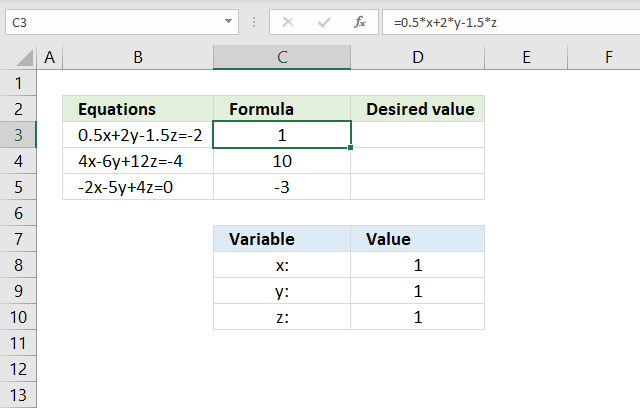The image above shows the formulas in cell C3, C4 and C5 respectively. They use the numbers in cell D8, D9 and D10 to return a calculated number.

The desired values in cell range D3:D5 correspond to the numbers in the equations. Cell D3 contains -2, D4, contains -4 and cell D5 contains 0 (zero).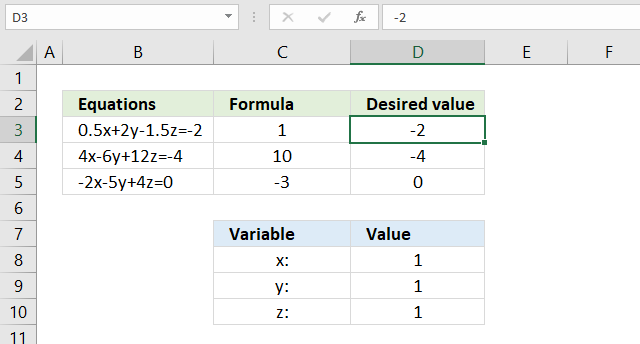### 1.1 How to install add-in Solver

1. Press with left mouse button on tab "File" on the ribbon.
2. Press with left mouse button on "Options". A dialog box appears.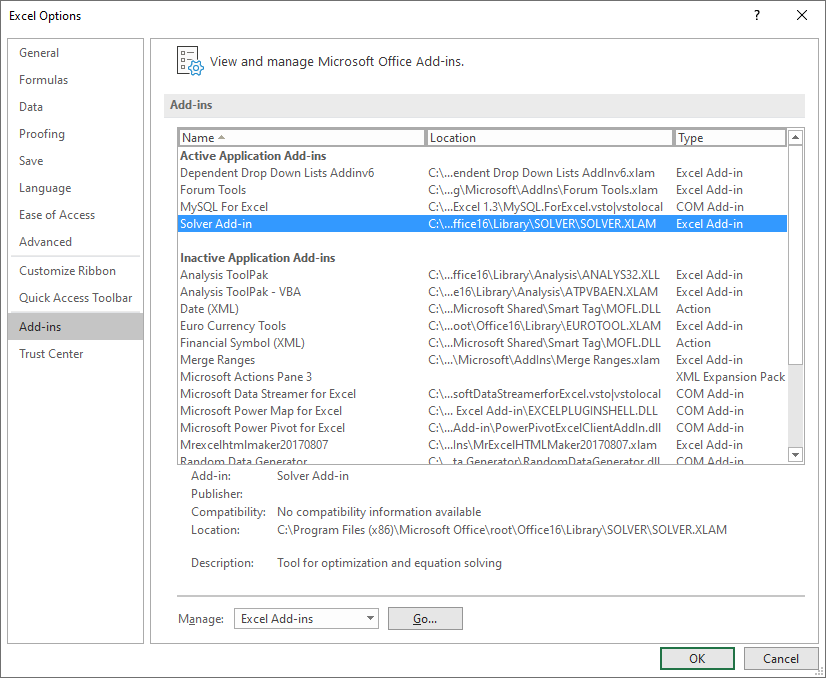3. Press with left mouse button on "Go..." button. Another dialog box appears.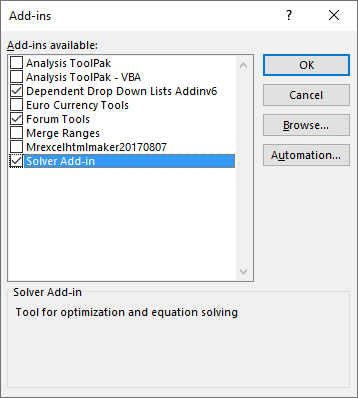4. Press with left mouse button on checkbox "Solver Add-In" to enable it.
5. Press with left mouse button on OK button.

Go to tab "Data" on the ribbon, the Solver button is now available usually on the right side of the ribbon.

### 1.2 Solver settings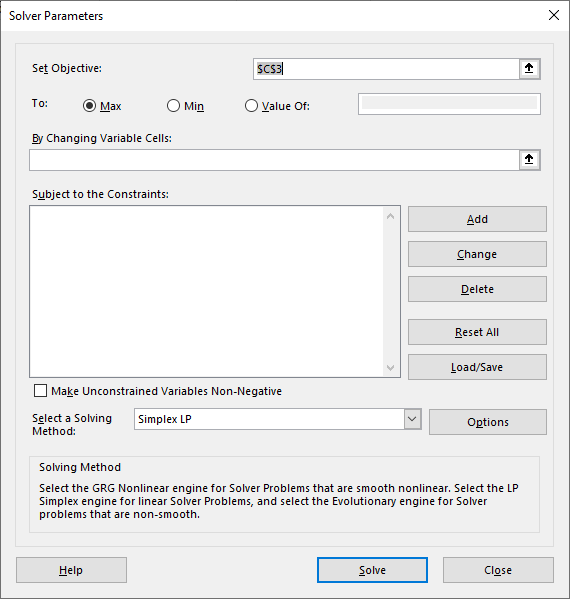Press with left mouse button on the Solver button on tab "Data" on the ribbon. A dialog box appears showing Solver settings that you can customize.

1. Press with mouse on arrow next to "Set Objective" in order to select a cell in the next step.
2. Press with mouse on cell C3.
3. Select the radio button "Value Of:" and type -2 in the field.
4. Press with mouse on the arrow that corresponds to "By changing Variable Cells:".
5. Select cell range D8:D10.
6. Press with mouse on "Add" button next to "Subject to the Constraints:". A dialog box appears.
7. Select Cell Reference C3.
8. Select the equal sign.
9. Select "Constraint:" D3. The dialog box now looks like this, see image below.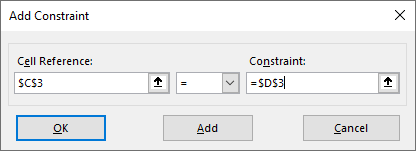10. Press with left mouse button on "OK" button.
11. Repeat steps 6 to 10 using cells C4 = D4 and C5 = D5.
12. Change "Select a Solving Method:" to "Simplex LP".

The Solver dialog box now looks like this, see image below.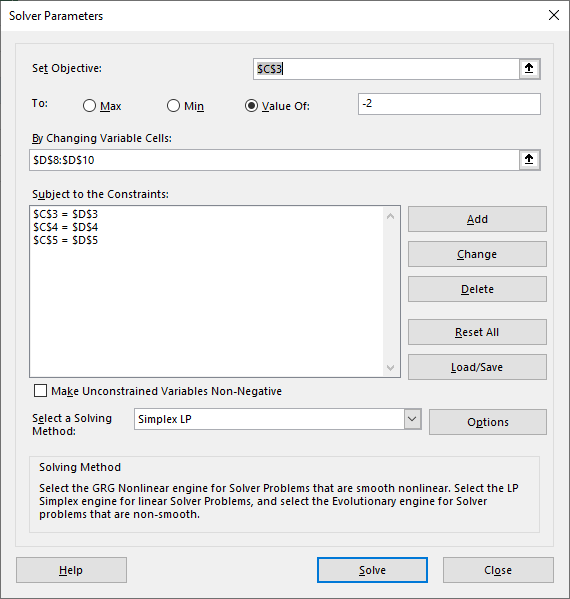Press with left mouse button on "Solve" button. The following dialog box appears if a solution is found.Deselect checkbox "Return to Solver Parameters Dialog" if you are happy with the solution. Press with left mouse button on "OK" button to dismiss the dialog box.

## 2. Solve linear equations using Excel formulas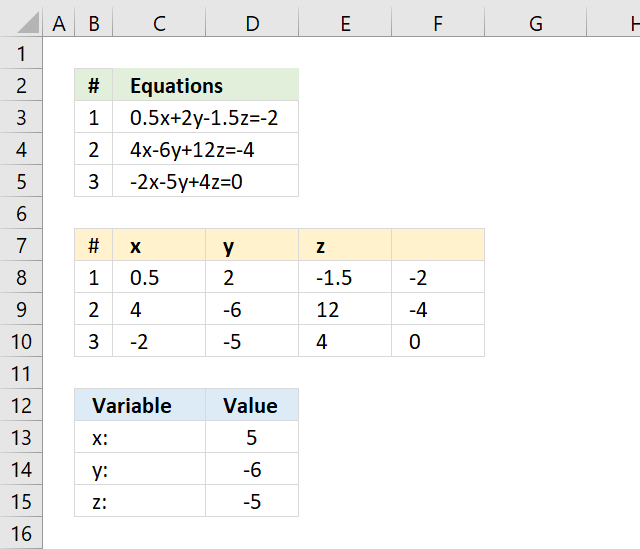The image above shows how to solve three simultaneous equations with three variables using one Excel formula. Cell range C3:C5 contains three equations with variables x, y and z.

First, extract the numbers from these three equations. The number before the x variable in the first equation goes to cell C8, in this example 0.5 is entered in cell C8.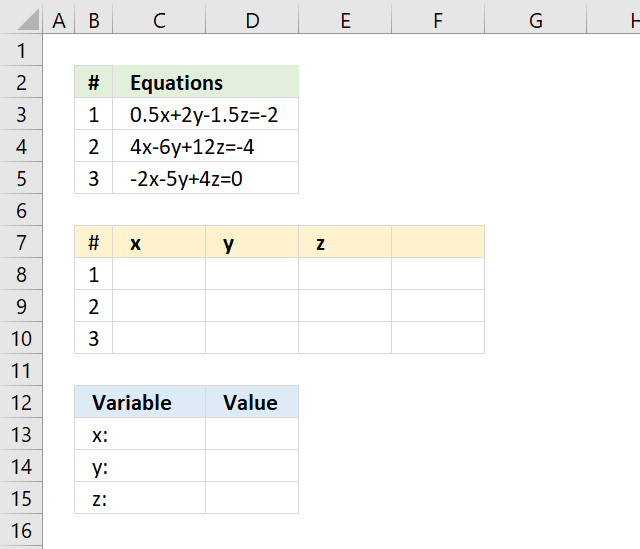Continue with the second equation which is 4, enter 4 in cell C9. Continue with the remaining variables and equations until all numbers have been extracted.

The constants are entered in cell range F8:F10. See the image below.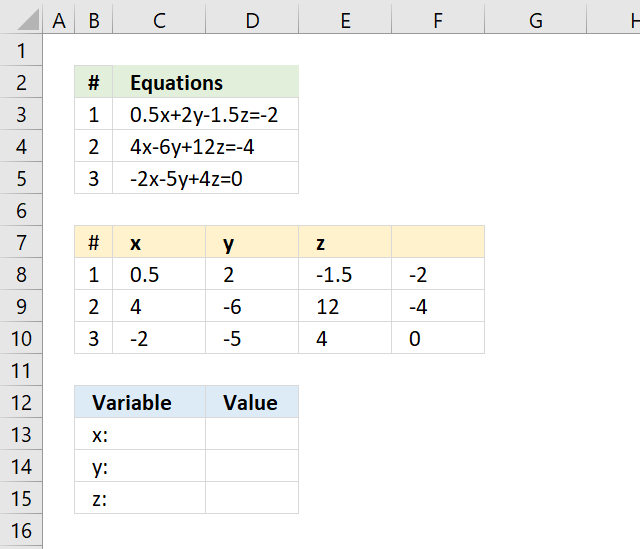Now you need to enter the following array formula in cell D13.

Array formula in cell D13:D15:

=MMULT(MINVERSE(C8:E10), F8:F10)

Excel 365 subscribers enter the formula in cell D13 and press enter. The formula is a dynamic array formula and the result is an array of numbers that Excel spills to cells below if empty.

You need to enter the formula as an array formula in cell range D13:D15 if you own an earlier Excel version, here are the steps.

1. Select cell range D13:D15.
2. Copy above formula and paste to the formula bar, see image below.
3. Press and hold CTRL and SHIFT keys simultaneously.
4. Press Enter once.
5. Release CTRL and SHIFT keys.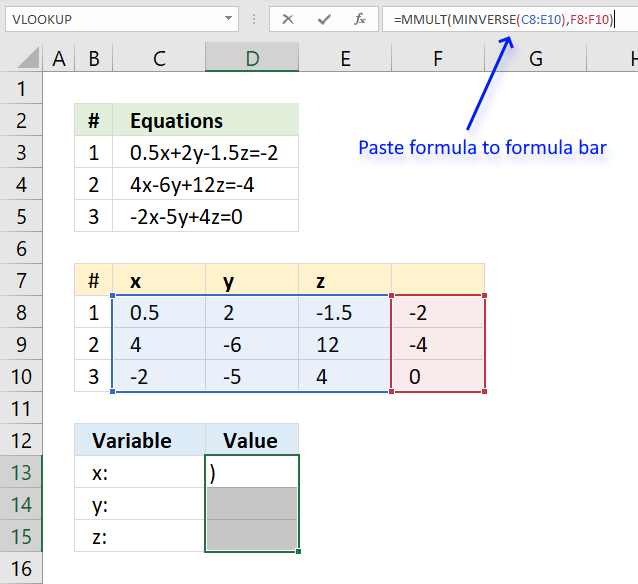The formula bar now shows a beginning and ending curly brackets if you successfully entered the array formula, see image below.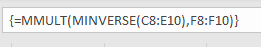The image below shows that cells D13, D14 and D15 now contain numbers returned from the array formula we just entered.### Explaining formula in cell D13

The "Evaluate Formula" tool allows you to examine a formula calculation in greater detail. Select the cell containing the formula you want to examine. Go to tab "Formulas" on the ribbon, press with left mouse button on the "Evaluate formula" button.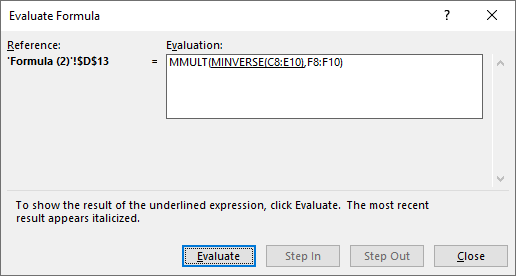A dialog box appears showing the formula, see image above. The underlined expression is what is about to be calculated when you press the "Evaluate" button. The italicized values are the results of the most recent calculation.

The video below explains in great detail how to solve a 3x3 matrix using a matrix equation.

#### Step 1 - Calculate the inverse matrix

The MINVERSE function calculates the inverse matrix for a given array. This webpage How to Find the Inverse of a 3x3 Matrix explains in great detail two methods to calculate the inverse of a matrix.

MINVERSE(C8:E10)

becomes

MINVERSE({0.5, 2, -1.5; 4, -6, 12; -2, -5, 4})

and returns

{-2.57142857142857, 0.0357142857142858, -1.07142857142857; 2.85714285714286, 0.0714285714285714, 0.857142857142857; 2.28571428571429, 0.107142857142857, 0.785714285714286}

or in fractions: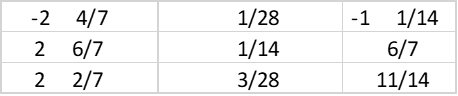#### Step 2 - Calculate the matrix product of two arrays

The MMULT function calculates the matrix product of two arrays.

MMULT(MINVERSE(C8:E10), F8:F10)

becomes

MMULT({-2.57142857142857, 0.0357142857142858, -1.07142857142857; 2.85714285714286, 0.0714285714285714, 0.857142857142857; 2.28571428571429, 0.107142857142857, 0.785714285714286}, F8:F10)

becomes

MMULT({-2.57142857142857,0.0357142857142858,-1.07142857142857;2.85714285714286,0.0714285714285714,0.857142857142857;2.28571428571429,0.107142857142857,0.785714285714286},{-2;-4;0})

and returns

{5; -6; -5}.

### Get the Excel fileSolving-simultaneous-linear-equationsv2.xlsx